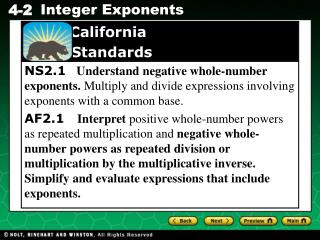# California Standards - PowerPoint PPT PresentationDownload PresentationCalifornia Standards

Download Presentation## California Standards

- - - - - - - - - - - - - - - - - - - - - - - - - - - E N D - - - - - - - - - - - - - - - - - - - - - - - - - - -
##### Presentation Transcript

1. California Standards NS2.1 Understand negative whole-number exponents. Multiply and divide expressions involving exponents with a common base. AF2.1 Interpret positive whole-number powers as repeated multiplication and negative whole-number powers as repeated division or multiplication by the multiplicative inverse. Simplify and evaluate expressions that include exponents.

2. 1 1 –1 10 = 10–2 = 10 10 • 10 1 = = 0.1 1 10 = 0.01 = 100 Additional Example 1: Using a Pattern to Evaluate Negative Exponents Simplify the powers of 10. A. 10–2 Multiply. Write as a decimal. B. 10–1 Multiply. Write as a decimal.

3. 1 10–8 = 10 • 10 • 10 • 10 • 10 • 10 • 10 • 10 1 = 100,000,000 Check It Out! Example 1A Simplify the powers of 10. 10–8 Multiply. Write as a decimal. = 0.00000001

4. 1 10–9 = 10 • 10 • 10 • 10 • 10 • 10 • 10 • 10 • 10 1 = 1,000,000,000 Check It Out! Example 1B Simplify the powers of 10. 10–9 Extend the pattern from Example 1A. Multiply. Write as a decimal. = 0.000000001

5. NEGATIVE EXPONENTS

6. 1 Find the product. = 5 • 5 • 5 1 = 125 Additional Example 2A: Evaluating Negative Exponents Simplify. 5–3 Write the power under 1; change the sign of the exponent. Simplify.

7. 1 Find the product. = –10 • –10 • –10 1 = –1000 Additional Example 2B: Evaluating Negative Exponents Simplify. (–10)–3 Write the power under 1; change the sign of the exponent. Simplify. = –0.001

8. 1 Find the product. = 4 • 4 1 = 16 Check It Out! Example 2A Simplify. 4–2 Write the power under 1; change the sign of the exponent. Simplify.

9. 1 Find the product. = –7 • –7 • –7 • –7 1 = 2401 Check It Out! Example 2B Simplify. (–7)–4 Write the power under 1; change the sign of the exponent. Simplify.

10. 1 23 1 8 7 8 5 5 – + 1 5 – + 1 Additional Example 3: Using the Order of Operations Simplify 5 – (6 – 4)–3+ (–2)0. 5 – (6 – 4)–3+ (–2)0 5 – (2)–3+ (–2)0 Subtract inside the parentheses. Rewrite 2-3. Simplify the powers. Add and subtract from left to right.